10万元也能玩科技？选TA就对了

2019年10月10日 13:19 来源：车行天下 超过：10679次关注

 如今的汽车市场已被“年轻人”所主导，各个车企纷纷使出浑身解数，来吸引这群新鲜血液。今年，在第二代逸动、第二代逸动XT相继上市后，长安再次拿出一款诚意之作——第二代逸动高能版，从9月20日刚刚结束的发布会现场来看，这很显然是迎合热血青年而推出了一款车型。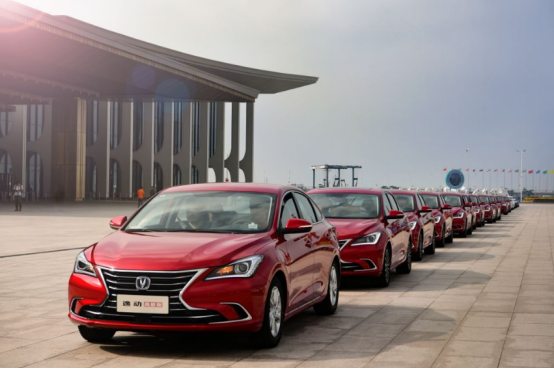本次推出的第二代逸动高能版共计十款车型，其中八款为燃油车型，从低到高分别为锐锋型、先锋型、争锋型、领锋型， 另外还配有手动和自动的48V蓝动版“劲锋型”车型。     相较于第二代逸动，逸动高能版在动力上没有变化，依旧沿用了Blue Core 1.6L GDI缸内直喷全铝合金发动机，最大功率为94千瓦，峰值扭矩达到168 N·m。与发动机匹配的是爱信6AT变速箱。那么既然动力系统并没有改变，那么这所谓的“高能版”究竟又哪些升级之处呢？     外观升级     高能版车型新增犀利造型的LED前大灯，亮度与节能效果出众，精确照射前路，保证驾驶最佳视野，时刻散发摄人魅力。另外，激流式LED日间行车灯及LED后位置灯，耀目动魄展露不凡，所过之处皆惊艳。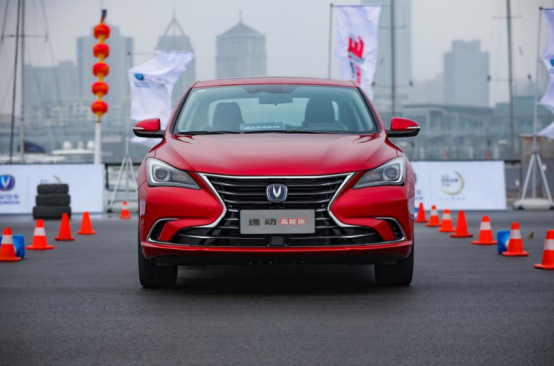除此之外，全新双色多幅设计的17英寸动感刀锋式轮毂，也在无形中展露更为动感和凌厉的气势。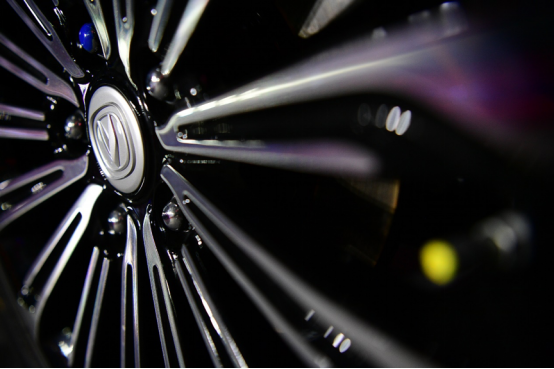配置全面升级     10.25英寸高清全液晶仪表+10.25英寸中控高清液晶显示屏，双10.25英寸屏幕之间，可以实现导航、音乐、电话等多种丰富的信息交互。并创造性地采用了“飞屏功能”，您可以轻松通过“三指左滑”的手势，将信息从中控屏“飞”到液晶仪表盘上，在专注前方的过程中，仅用余光即可轻松看到信息，大大提高行车安全。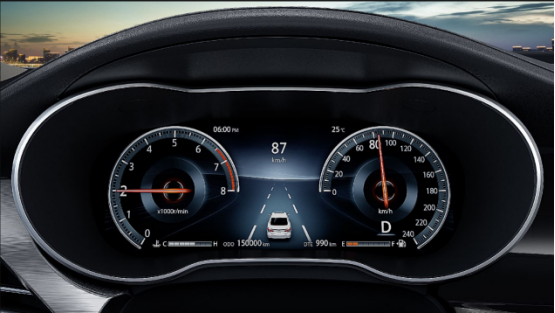令人惊喜的是，逸动高能版在智能化配置上方面也绝对“高能”。除了搭载了全新升级的inCall 3.0智能互联系统外，还做到了8万级首搭PAB预警辅助制动系统、ACC全速自适应巡航系统，以及OTA远程升级、TPMA胎压监测等贴心便利配置，让消费者的用车生活变得更加人性智能。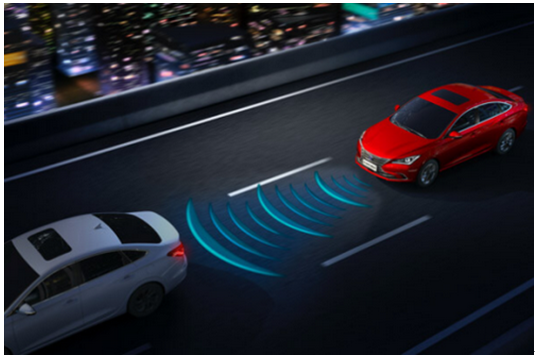除此之外，第二代逸动高能版从用户角度出发，对消费者更加重视的驾乘感受进行调整和升级，全新升级的“小安，你好”智能语音系统，语音控制能力大幅提升；自带全时4G在线，基础流量终身免费；搭载后排中央头枕，可触可感的舒适设计，随时照顾你的坐姿，舒享每段旅程。另外，还拥有了带记忆功能的电动六向座椅，给用户带来尊贵的迎宾体验。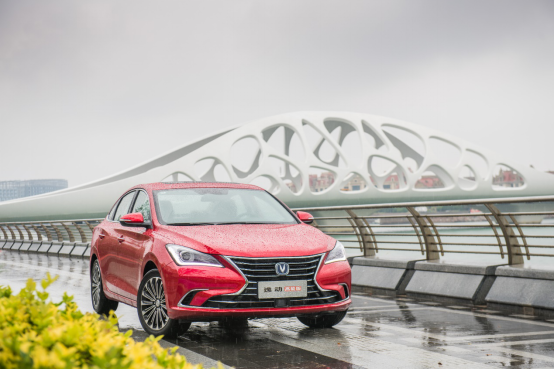逸动，作为长安汽车在2012年推出的匠心之作，拥有辉煌的发展历史，逸动系列至今用户已近80万，是中国品牌汽车发展的里程碑式产品。最近，热门综艺《这！就是灌篮》引爆网络，作为官方用车的长安第二代逸动可谓大放异彩，不仅以颜值吸引了一大批观众，还在节目中玩起了花式植入。邀请节目中两位人气选手挑战“隔窗传球”，利用第二代逸动车身的搭载ACC全速自适应巡航系统，实现车辆匀速前进，以此体现第二代逸动的高能实力。这些受到人们关注的品牌活动，正是俘获年轻消费者、激发品牌活力的重要一步。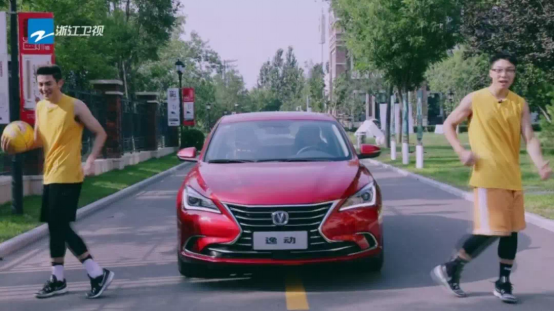随着第二代逸动的荣耀上市和第二代逸动高能版的蓄势待发，逸动城市任务战火重燃，    10月12日，2018年逸动城市任务即将登陆成都。此次城市任务以青岛站为起点，还将在成都、苏州、郑州等全国范围内举办近500场逸动城市任务。而且2018年逸动城市任务打破常规，以跨界形式与篮球IP衍生的竞技类节目《这！就是灌篮》融合，全程植入灌篮元素，借篮球狂欢的“青春、热血、励志”正能量传达逸动系列“高效、活力、非凡、乐观”的品牌精神，强化了第二代逸动领先的智能化配置以及优秀的动态体验。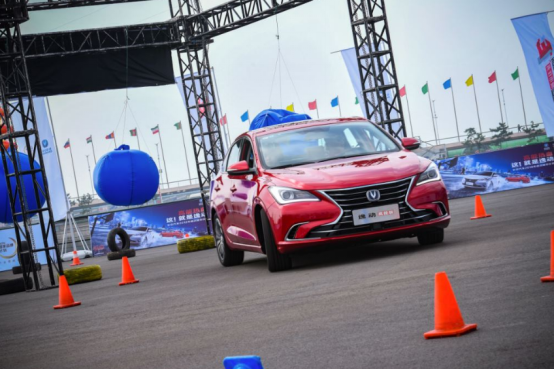逸动城市任务，是长安汽车为逸动品牌和用户精心量身定制的创新体验式品牌活动，2013-2015年，逸动作为城市任务的主角，以“竞技”为核心，将拼搏精神融入活动，塑造了积极向上的品牌形象，数万余用户参与到活动中。     第二代逸动高能版通过“高能”的产品实力和不断贯彻“高效、活力、乐观、非凡”的品牌精神，为广大消费者带来更高一级的产品体验，也难怪能在竞争激烈的汽车圈子里畅销多年，圈粉无数了。总体来看，逸动高能版在同级别车型中的确有着不俗的实力。此次第二代逸动高能版的上市，势必会助力销量的提升。同时年轻消费者可用最实惠的价格买到拥有更具竞争力的车型，正所谓一举两得。

相关文章

0-500 字已有评论 0条 查看评论>>

﻿
• 快速找车
• 选择品牌
• 选择品牌
• A  奥迪
• A  阿斯顿·马丁
• A  阿尔法·罗密欧
• B  宝沃
• B  布加迪
• B  巴博斯
• B  保时捷
• B  宾利
• B  奔驰
• B  宝马
• B  本田
• B  别克
• B  标致
• B  比亚迪
• B  宝骏
• B  北汽制造
• B  北汽新能源
• B  北汽幻速
• B  北汽威旺
• B  北京汽车
• B  奔腾
• B  北汽绅宝
• C  长安
• C  长安商用
• C  长城
• C  昌河
• D  大众
• D  道奇
• D  DS
• D  东南
• D  东风风神
• D  东风风行
• D  东风小康
• D  东风风度
• D  东风
• F  福特
• F  丰田
• F  菲亚特
• F  法拉利
• F  福田
• F  福迪
• F  福汽启腾
• G  观致
• G  广汽传祺
• G  广汽吉奥
• G  GMC
• H  红旗
• H  汉腾汽车
• H  哈弗
• H  哈飞
• H  海格
• H  海马
• H  华颂
• H  黄海
• H  华泰
• H  恒天
• J  吉利汽车
• J  捷豹
• J  Jeep
• J  江淮
• J  江铃
• J  金杯
• J  九龙
• J  金旅
• K  凯翼
• K  凯迪拉克
• K  克莱斯勒
• K  科尼塞克
• K  卡威
• K  开瑞
• L  路虎
• L  林肯
• L  劳斯莱斯
• L  兰博基尼
• L  雷克萨斯
• L  铃木
• L  雷诺
• L  理念
• L  力帆
• L  莲花汽车
• L  猎豹
• L  路特斯
• L  陆风
• M  马自达
• M  MG
• M  MINI
• M  玛莎拉蒂
• M  摩根
• M  迈凯轮
• N  纳智捷
• O  欧宝
• O  讴歌
• O  欧朗
• Q  奇瑞
• Q  起亚
• Q  启辰
• R  日产
• R  荣威
• R  瑞麒
• S  三菱
• S  斯威汽车
• S  萨博
• S  smart
• S  斯柯达
• S  斯巴鲁
• S  思铭
• S  双龙
• S  上汽大通
• S  双环
• T  特斯拉
• T  腾势
• W  沃尔沃
• W  五菱汽车
• W  五十铃
• W  威兹曼
• W  威麟
• X  现代
• X  雪佛兰
• X  雪铁龙
• X  西雅特
• Y  一汽
• Y  英菲尼迪
• Y  英致
• Y  依维柯
• Y  野马汽车
• Y  永源
• Z  众泰
• Z  中华
• Z  中兴
• Z  知豆
• 选择车系
• 选择车系
• 车型对比
• 选择品牌
• 选择品牌
• A  奥迪
• A  阿斯顿·马丁
• A  阿尔法·罗密欧
• B  宝沃
• B  布加迪
• B  巴博斯
• B  保时捷
• B  宾利
• B  奔驰
• B  宝马
• B  本田
• B  别克
• B  标致
• B  比亚迪
• B  宝骏
• B  北汽制造
• B  北汽新能源
• B  北汽幻速
• B  北汽威旺
• B  北京汽车
• B  奔腾
• B  北汽绅宝
• C  长安
• C  长安商用
• C  长城
• C  昌河
• D  大众
• D  道奇
• D  DS
• D  东南
• D  东风风神
• D  东风风行
• D  东风小康
• D  东风风度
• D  东风
• F  福特
• F  丰田
• F  菲亚特
• F  法拉利
• F  福田
• F  福迪
• F  福汽启腾
• G  观致
• G  广汽传祺
• G  广汽吉奥
• G  GMC
• H  红旗
• H  汉腾汽车
• H  哈弗
• H  哈飞
• H  海格
• H  海马
• H  华颂
• H  黄海
• H  华泰
• H  恒天
• J  吉利汽车
• J  捷豹
• J  Jeep
• J  江淮
• J  江铃
• J  金杯
• J  九龙
• J  金旅
• K  凯翼
• K  凯迪拉克
• K  克莱斯勒
• K  科尼塞克
• K  卡威
• K  开瑞
• L  路虎
• L  林肯
• L  劳斯莱斯
• L  兰博基尼
• L  雷克萨斯
• L  铃木
• L  雷诺
• L  理念
• L  力帆
• L  莲花汽车
• L  猎豹
• L  路特斯
• L  陆风
• M  马自达
• M  MG
• M  MINI
• M  玛莎拉蒂
• M  摩根
• M  迈凯轮
• N  纳智捷
• O  欧宝
• O  讴歌
• O  欧朗
• Q  奇瑞
• Q  起亚
• Q  启辰
• R  日产
• R  荣威
• R  瑞麒
• S  三菱
• S  斯威汽车
• S  萨博
• S  smart
• S  斯柯达
• S  斯巴鲁
• S  思铭
• S  双龙
• S  上汽大通
• S  双环
• T  特斯拉
• T  腾势
• W  沃尔沃
• W  五菱汽车
• W  五十铃
• W  威兹曼
• W  威麟
• X  现代
• X  雪佛兰
• X  雪铁龙
• X  西雅特
• Y  一汽
• Y  英菲尼迪
• Y  英致
• Y  依维柯
• Y  野马汽车
• Y  永源
• Z  众泰
• Z  中华
• Z  中兴
• Z  知豆
• 选择车系
• 选择车系
• 选择车型
• 选择车型
• 意见反馈